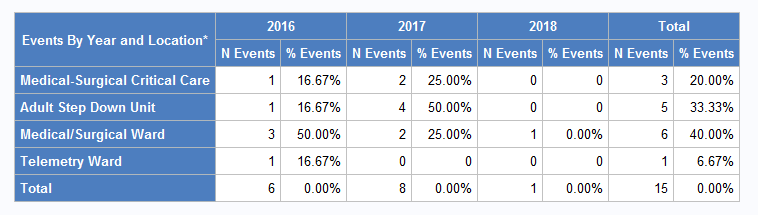## proc tabulate incorrect column percentages

I am using proc tabulate to create a table like the one below.It is intended to count the number of observations for each hospital location by year. Additionally, I would like to generate column percentages for each year's observations.

I was able to create my desired layout, but my column percentage totals are coming out to 0%. They should be 100%. I can't seem to figure out what has happened with my nesting to cause this error. Any assistance would be greatly appreciated!

This is the code that I am using:

proc tabulate data=mydata ;
CLASSLEV location specdateYR/ S=[background=bigb foreground=white];
TABLE location = ' ' (ALL = {label='Total' S=[background=bigb foreground=white]}),
(specdateYR = ' ' (All= {label='Total' S=[background=bigb foreground=white]}))
*((N= {label='N Events' S=[background=bigb foreground=white]})
((colpctn= {label='% Events' S=[background=bigb foreground=white]})*f=pctfmt.))
/misstext='0' BOX=[label='Events By Year and Location*' S=[background=bigb foreground=white]];
run;

1 ACCEPTED SOLUTION

Accepted Solutions

## Re: proc tabulate incorrect column percentages

Show the definitions of your PCTFMT. Likely you didn't allocate the correct options to show 100.00%.

3 REPLIES 3

## Re: proc tabulate incorrect column percentages

Remove the format and are they still 0?

## Re: proc tabulate incorrect column percentages

Show the definitions of your PCTFMT. Likely you didn't allocate the correct options to show 100.00%.

## Re: proc tabulate incorrect column percentages

This is the format I was using.

proc format;
picture pctfmt (round) other='09.99%';
run;

I realized that my format did not allow for 3 digits. I changed the format to

proc format;
picture pctfmt (round) other='009.99%';
run;

which corrected the issue.

Thank you for pointing me in the right direction!

Discussion stats
• 3 replies
• 665 views
• 0 likes
• 3 in conversation